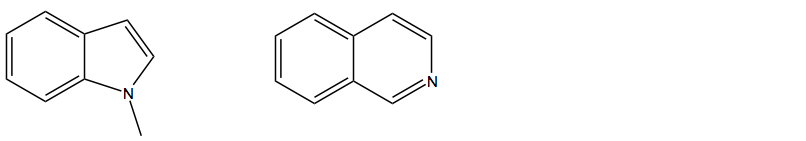Problem: For the following pair of compounds, identify which compound is the stronger base (circle one).

FREE Expert Solution
100% (113 ratings)
Problem Details

For the following pair of compounds, identify which compound is the stronger base (circle one).What scientific concept do you need to know in order to solve this problem?

Our tutors have indicated that to solve this problem you will need to apply the Basicity of Aromatic Heterocycles concept. You can view video lessons to learn Basicity of Aromatic Heterocycles Or if you need more Basicity of Aromatic Heterocycles practice, you can also practice Basicity of Aromatic Heterocycles practice problems .

What is the difficulty of this problem?

Our tutors rated the difficulty of For the following pair of compounds, identify which compound... as low difficulty.

How long does this problem take to solve?

Our expert Organic tutor, Jonathan took 2 minutes to solve this problem. You can follow their steps in the video explanation above.

What professor is this problem relevant for?

Based on our data, we think this problem is relevant for Professor Shanz's class at GS.